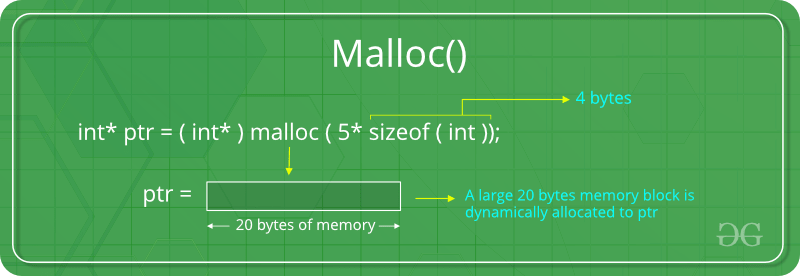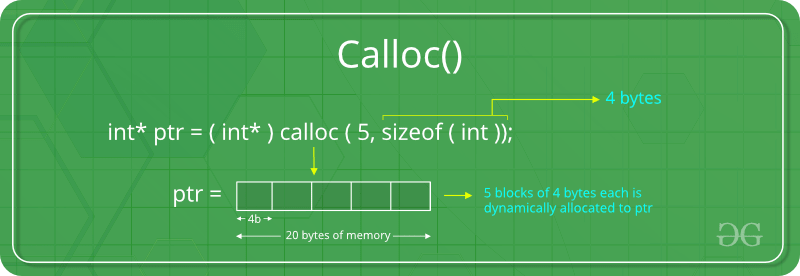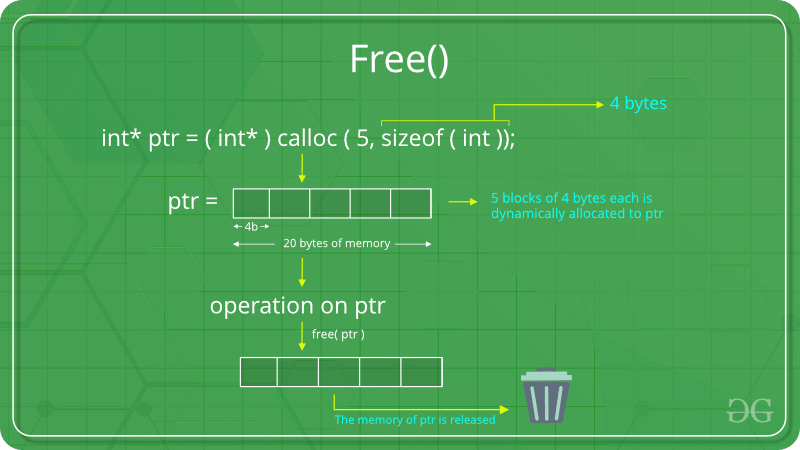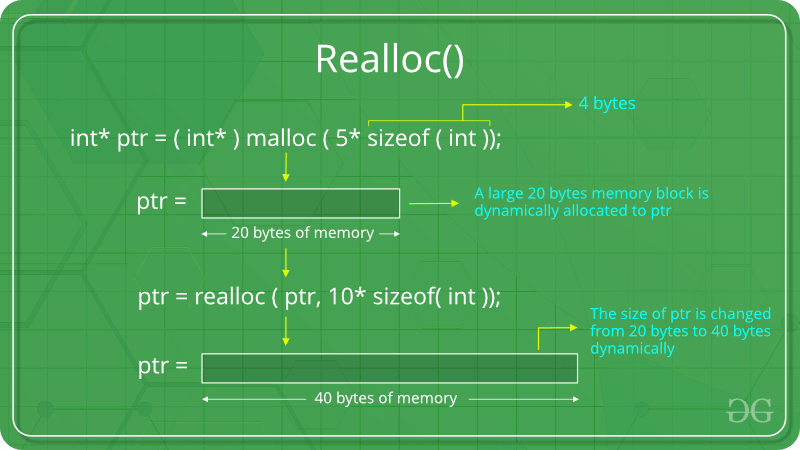# Dynamic Memory Allocation in C using malloc(), calloc(), free() and realloc()

Since C is a structured language, it has some fixed rules for programming. One of it includes changing the size of an array. An array is collection of items stored at continuous memory locations.As it can be seen that the length (size) of the array above made is 9. But what if there is a requirement to change this length (size). For Example,

• If there is a situation where only 5 elements are needed to be entered in this array. In this case, the remaining 4 indices are just wasting memory in this array. So there is a requirement to lessen the length (size) of the array from 9 to 5.
• Take another situation. In this, there is an array of 9 elements with all 9 indices filled. But there is a need to enter 3 more elements in this array. In this case 3 indices more are required. So the length (size) of the array needs to be changed from 9 to 12.

This procedure is referred to as Dynamic Memory Allocation in C.

Therefore, C Dynamic Memory Allocation can be defined as a procedure in which the size of a data structure (like Array) is changed during the runtime.

C provides some functions to achieve these tasks. There are 4 library functions provided by C defined under <stdlib.h> header file to facilitate dynamic memory allocation in C programming. They are:

1. malloc()
2. calloc()
3. free()
4. realloc()

Let’s look at each of them in greater detail.

1. ### C malloc() method

“malloc” or “memory allocation” method in C is used to dynamically allocate a single large block of memory with the specified size. It returns a pointer of type void which can be cast into a pointer of any form. It initializes each block with default garbage value.

Syntax:

`ptr = (cast-type*) malloc(byte-size)`

For Example:

ptr = (int*) malloc(100 * sizeof(int));

Since the size of int is 4 bytes, this statement will allocate 400 bytes of memory. And, the pointer ptr holds the address of the first byte in the allocated memory.If space is insufficient, allocation fails and returns a NULL pointer.

Example:

 `#include ` `#include ` ` `  `int` `main() ` `{ ` ` `  `    ``// This pointer will hold the ` `    ``// base address of the block created ` `    ``int``* ptr; ` `    ``int` `n, i; ` ` `  `    ``// Get the number of elements for the array ` `    ``n = 5; ` `    ``printf``(``"Enter number of elements: %d\n"``, n); ` ` `  `    ``// Dynamically allocate memory using malloc() ` `    ``ptr = (``int``*)``malloc``(n * ``sizeof``(``int``)); ` ` `  `    ``// Check if the memory has been successfully ` `    ``// allocated by malloc or not ` `    ``if` `(ptr == NULL) { ` `        ``printf``(``"Memory not allocated.\n"``); ` `        ``exit``(0); ` `    ``} ` `    ``else` `{ ` ` `  `        ``// Memory has been successfully allocated ` `        ``printf``(``"Memory successfully allocated using malloc.\n"``); ` ` `  `        ``// Get the elements of the array ` `        ``for` `(i = 0; i < n; ++i) { ` `            ``ptr[i] = i + 1; ` `        ``} ` ` `  `        ``// Print the elements of the array ` `        ``printf``(``"The elements of the array are: "``); ` `        ``for` `(i = 0; i < n; ++i) { ` `            ``printf``(``"%d, "``, ptr[i]); ` `        ``} ` `    ``} ` ` `  `    ``return` `0; ` `} `

Output:

```Enter number of elements: 5
Memory successfully allocated using malloc.
The elements of the array are: 1, 2, 3, 4, 5,
```
2. ### C calloc() method

“calloc” or “contiguous allocation” method in C is used to dynamically allocate the specified number of blocks of memory of the specified type. It initializes each block with a default value ‘0’.

Syntax:

`ptr = (cast-type*)calloc(n, element-size);`

For Example:

ptr = (float*) calloc(25, sizeof(float));

This statement allocates contiguous space in memory for 25 elements each with the size of the float.If space is insufficient, allocation fails and returns a NULL pointer.

Example:

 `#include ` `#include ` ` `  `int` `main() ` `{ ` ` `  `    ``// This pointer will hold the ` `    ``// base address of the block created ` `    ``int``* ptr; ` `    ``int` `n, i; ` ` `  `    ``// Get the number of elements for the array ` `    ``n = 5; ` `    ``printf``(``"Enter number of elements: %d\n"``, n); ` ` `  `    ``// Dynamically allocate memory using calloc() ` `    ``ptr = (``int``*)``calloc``(n, ``sizeof``(``int``)); ` ` `  `    ``// Check if the memory has been successfully ` `    ``// allocated by calloc or not ` `    ``if` `(ptr == NULL) { ` `        ``printf``(``"Memory not allocated.\n"``); ` `        ``exit``(0); ` `    ``} ` `    ``else` `{ ` ` `  `        ``// Memory has been successfully allocated ` `        ``printf``(``"Memory successfully allocated using calloc.\n"``); ` ` `  `        ``// Get the elements of the array ` `        ``for` `(i = 0; i < n; ++i) { ` `            ``ptr[i] = i + 1; ` `        ``} ` ` `  `        ``// Print the elements of the array ` `        ``printf``(``"The elements of the array are: "``); ` `        ``for` `(i = 0; i < n; ++i) { ` `            ``printf``(``"%d, "``, ptr[i]); ` `        ``} ` `    ``} ` ` `  `    ``return` `0; ` `} `

Output:

```Enter number of elements: 5
Memory successfully allocated using calloc.
The elements of the array are: 1, 2, 3, 4, 5,
```
3. ### C free() method

“free” method in C is used to dynamically de-allocate the memory. The memory allocated using functions malloc() and calloc() is not de-allocated on their own. Hence the free() method is used, whenever the dynamic memory allocation takes place. It helps to reduce wastage of memory by freeing it.

Syntax:

`free(ptr);`Example:

 `#include ` `#include ` ` `  `int` `main() ` `{ ` ` `  `    ``// This pointer will hold the ` `    ``// base address of the block created ` `    ``int` `*ptr, *ptr1; ` `    ``int` `n, i; ` ` `  `    ``// Get the number of elements for the array ` `    ``n = 5; ` `    ``printf``(``"Enter number of elements: %d\n"``, n); ` ` `  `    ``// Dynamically allocate memory using malloc() ` `    ``ptr = (``int``*)``malloc``(n * ``sizeof``(``int``)); ` ` `  `    ``// Dynamically allocate memory using calloc() ` `    ``ptr1 = (``int``*)``calloc``(n, ``sizeof``(``int``)); ` ` `  `    ``// Check if the memory has been successfully ` `    ``// allocated by malloc or not ` `    ``if` `(ptr == NULL || ptr1 == NULL) { ` `        ``printf``(``"Memory not allocated.\n"``); ` `        ``exit``(0); ` `    ``} ` `    ``else` `{ ` ` `  `        ``// Memory has been successfully allocated ` `        ``printf``(``"Memory successfully allocated using malloc.\n"``); ` ` `  `        ``// Free the memory ` `        ``free``(ptr); ` `        ``printf``(``"Malloc Memory successfully freed.\n"``); ` ` `  `        ``// Memory has been successfully allocated ` `        ``printf``(``"\nMemory successfully allocated using calloc.\n"``); ` ` `  `        ``// Free the memory ` `        ``free``(ptr1); ` `        ``printf``(``"Calloc Memory successfully freed.\n"``); ` `    ``} ` ` `  `    ``return` `0; ` `} `

Output:

```Enter number of elements: 5
Memory successfully allocated using malloc.
Malloc Memory successfully freed.

Memory successfully allocated using calloc.
Calloc Memory successfully freed.
```
4. ### C realloc() method

“realloc” or “re-allocation” method in C is used to dynamically change the memory allocation of a previously allocated memory. In other words, if the memory previously allocated with the help of malloc or calloc is insufficient, realloc can be used to dynamically re-allocate memory. re-allocation of memory maintains the already present value and new blocks will be initialized with default garbage value.

Syntax:

```ptr = realloc(ptr, newSize);

where ptr is reallocated with new size 'newSize'.
```If space is insufficient, allocation fails and returns a NULL pointer.

Example:

 `#include ` `#include ` ` `  `int` `main() ` `{ ` ` `  `    ``// This pointer will hold the ` `    ``// base address of the block created ` `    ``int``* ptr; ` `    ``int` `n, i; ` ` `  `    ``// Get the number of elements for the array ` `    ``n = 5; ` `    ``printf``(``"Enter number of elements: %d\n"``, n); ` ` `  `    ``// Dynamically allocate memory using calloc() ` `    ``ptr = (``int``*)``calloc``(n, ``sizeof``(``int``)); ` ` `  `    ``// Check if the memory has been successfully ` `    ``// allocated by malloc or not ` `    ``if` `(ptr == NULL) { ` `        ``printf``(``"Memory not allocated.\n"``); ` `        ``exit``(0); ` `    ``} ` `    ``else` `{ ` ` `  `        ``// Memory has been successfully allocated ` `        ``printf``(``"Memory successfully allocated using calloc.\n"``); ` ` `  `        ``// Get the elements of the array ` `        ``for` `(i = 0; i < n; ++i) { ` `            ``ptr[i] = i + 1; ` `        ``} ` ` `  `        ``// Print the elements of the array ` `        ``printf``(``"The elements of the array are: "``); ` `        ``for` `(i = 0; i < n; ++i) { ` `            ``printf``(``"%d, "``, ptr[i]); ` `        ``} ` ` `  `        ``// Get the new size for the array ` `        ``n = 10; ` `        ``printf``(``"\n\nEnter the new size of the array: %d\n"``, n); ` ` `  `        ``// Dynamically re-allocate memory using realloc() ` `        ``ptr = ``realloc``(ptr, n * ``sizeof``(``int``)); ` ` `  `        ``// Memory has been successfully allocated ` `        ``printf``(``"Memory successfully re-allocated using realloc.\n"``); ` ` `  `        ``// Get the new elements of the array ` `        ``for` `(i = 5; i < n; ++i) { ` `            ``ptr[i] = i + 1; ` `        ``} ` ` `  `        ``// Print the elements of the array ` `        ``printf``(``"The elements of the array are: "``); ` `        ``for` `(i = 0; i < n; ++i) { ` `            ``printf``(``"%d, "``, ptr[i]); ` `        ``} ` ` `  `        ``free``(ptr); ` `    ``} ` ` `  `    ``return` `0; ` `} `

Output:

```Enter number of elements: 5
Memory successfully allocated using calloc.
The elements of the array are: 1, 2, 3, 4, 5,

Enter the new size of the array: 10
Memory successfully re-allocated using realloc.
The elements of the array are: 1, 2, 3, 4, 5, 6, 7, 8, 9, 10,
```

Attention reader! Don’t stop learning now. Get hold of all the important DSA concepts with the DSA Self Paced Course at a student-friendly price and become industry ready.

My Personal Notes arrow_drop_upContent Manager at LambdaTest

If you like GeeksforGeeks and would like to contribute, you can also write an article using contribute.geeksforgeeks.org or mail your article to contribute@geeksforgeeks.org. See your article appearing on the GeeksforGeeks main page and help other Geeks.

Please Improve this article if you find anything incorrect by clicking on the "Improve Article" button below.

Article Tags :
Practice Tags :

112

Please write to us at contribute@geeksforgeeks.org to report any issue with the above content.